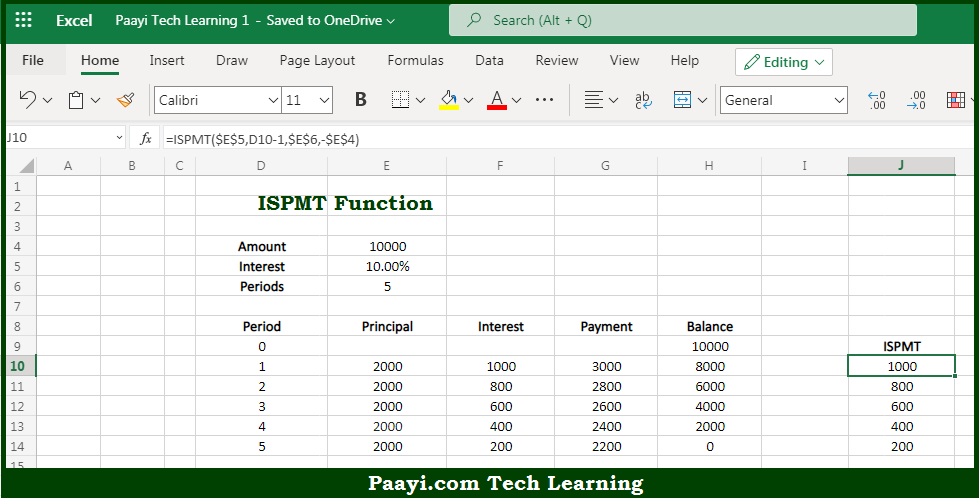# Learn How to UseMicrosoft Excel ISPMT Function

Written by | 0 Comments | 496 Views

In this article, you will learn how to use the Microsoft Excel ISPMT function and its prime function in Microsoft Excel. You will also get to know the Microsoft Excel ISPMT function return value and syntax with the help of some examples.

Microsoft Excel ISPMT Function

The main purpose of the Microsoft Excel ISPMT function is to get the interest paid for the specific period. That implies, with the help of ISPMT function you can able to calculate the interest paid during a given period of an investment where principal payments are equal. The given period is specified as a zero-based number instead of a 1-based number. So, with the help of ISPMT function, you can able to get the interest paid for the specific period.

Return Value of ISPMT Function

The return value will be the interest amount in a given period.

Syntax of ISPMT Function

=ISPMT(rate, per, nper, pv)

Where the arguments:

• rate: This is the interest rate.
• per: This is a period starting with 0, not 1.
• nper: This is the number of periods.
• pv: This is the present value.

## How to Use Microsoft Excel ISPMT Function?So we know that Microsoft Excel ISPMT function you can able to get the interest paid for the specific period. That implies, with the help of the ISPMT function you can able to calculate the interest paid during a given period of an investment where principal payments are equal. The given period is specified as a zero-based number instead of a 1-based number. So, with the help of the ISPMT function, you can able to get the interest paid for the specific period.